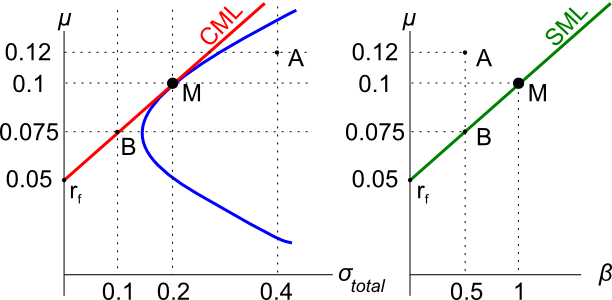# Fight Finance

#### CoursesTagsRandomAllRecentScoresAssets A, B, M and $r_f$ are shown on the graphs above. Asset M is the market portfolio and $r_f$ is the risk free yield on government bonds. Which of the below statements is NOT correct?

A graph of assets’ expected returns $(\mu)$ versus standard deviations $(\sigma)$ is given in the graph below. The CML is the capital market line.Which of the following statements about this graph, Markowitz portfolio theory and the Capital Asset Pricing Model (CAPM) theory is NOT correct?

Which of the following statements is NOT correct? Fairly-priced assets should:

Over-priced assets should NOT: